• # John Napier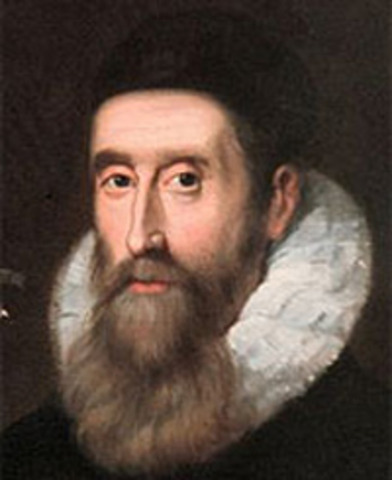Invented a system of moveable rods based on logarithms that could multiply, divide, and calculate square and cube roots. This is important since it was an advanvcement into more indepth math.
• # Wilhelm Schickard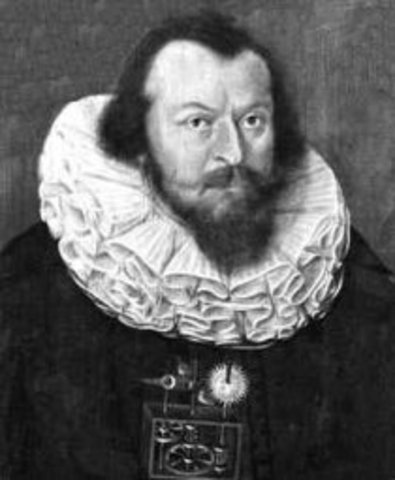Wilhelm Schickard Develops the First mechanical calculator. This is important since this is the first time a machine does the thinking on its own.
• # Phillip-Malthus Hahn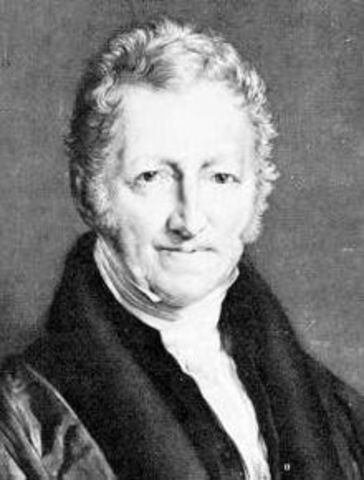The first calculating machines are sold. This is important since this was the first sales of computers.
• # Joseph-Marie Jacquard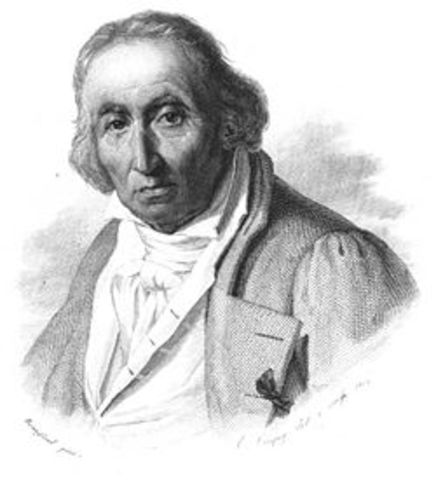Invented the first computer program which was a punch card that told a loom what to do. This is important since it was the first program to produce something instead of just calculate.
• # Arithmometer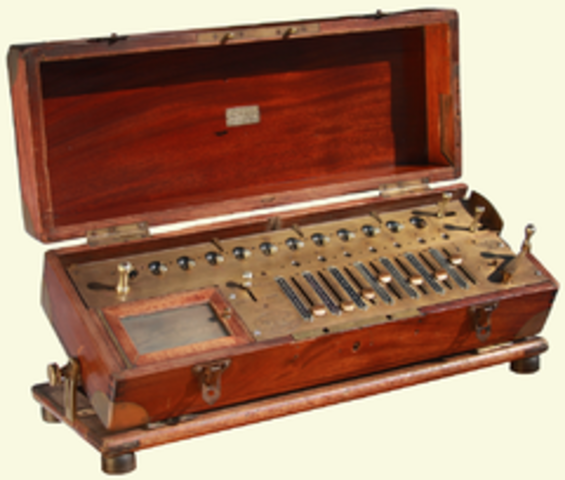The arithmometer was mass produced. This is important since this is where mass production started.
• # William Austin Burtthe first typwriter is invented. This is important since it's like the first google doc.
• # Konrad Zuse Binary punch tape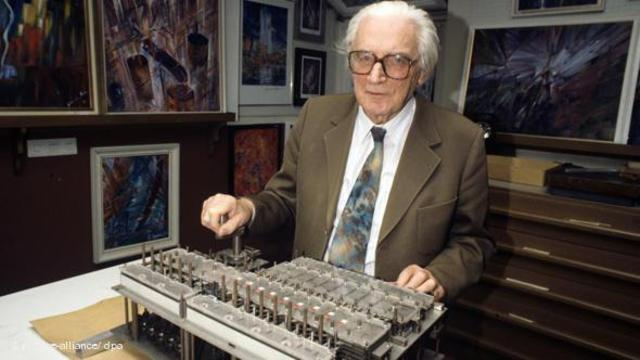The use of binary punch tape. This is important since most computers use binary.
• # Cathode Ray TubeThe cathode ray tube is invented as the forerunner to random-access memory. This important since most computer use RAM.
• # The first electronic computer is invented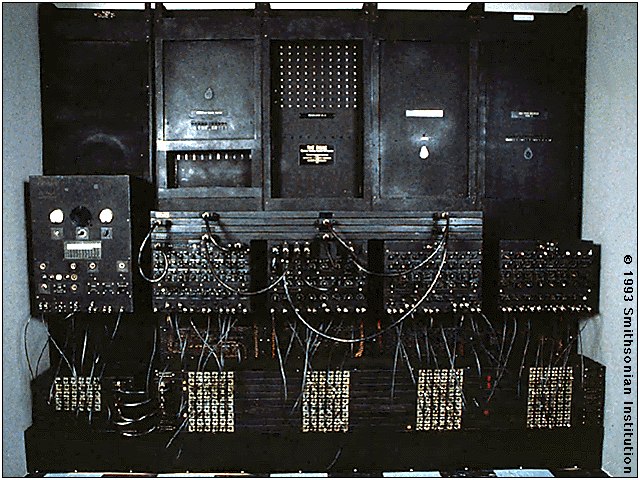Hideo Yamachito invented the first electronic computer. This is important since it is the first computer.
• # Arpanet is builtThis is the first network built by the military and is the first building block to the internet. This is important since this is how we communicate.